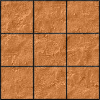#### You may also like### Paving the Way

A man paved a square courtyard and then decided that it was too small. He took up the tiles, bought 100 more and used them to pave another square courtyard. How many tiles did he use altogether?### Square Areas

Can you work out the area of the inner square and give an explanation of how you did it?### Chess

What would be the smallest number of moves needed to move a Knight from a chess set from one corner to the opposite corner of a 99 by 99 square board?

# Square Coordinates

##### Age 11 to 14 Challenge Level:

Play with this interactivity until you can draw tilted squares confidently.

Formulate and describe a general instruction for the construction of a square when you are given two adjacent corners.

Formulate and describe a general instruction for the construction of a square when you are given two opposite corners.

Decide whether any of the collections of points below form a square.
If so, which ones?
Can you do this without plotting the points on a grid?

1. $(8,3)$, $(7,8)$, $(2,7)$, $(3,2)$
2. $(3,3)$, $(7,4)$, $(8,8)$, $(4,7)$
3. $(16,19)$, $(18,22)$, $(21,20)$, $(19,17)$
4. $(4,20)$, $(21,19)$, $(20,2)$, $(3,3)$

Explain how you decided.